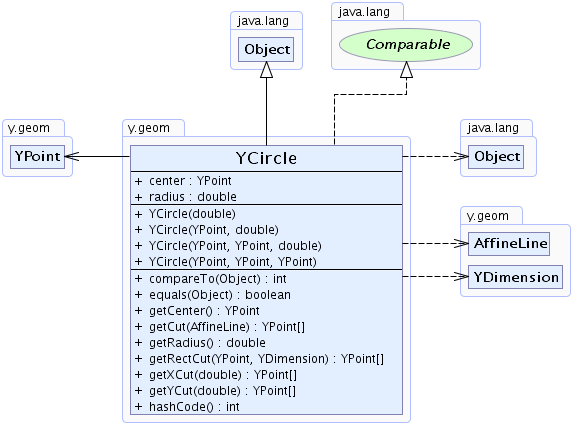Search this API

## y.geom Class YCircle

```java.lang.Objecty.geom.YCircle
```
All Implemented Interfaces:
java.lang.Comparable

`public class YCircleextends java.lang.Objectimplements java.lang.Comparable`

This class represents a circle in the plane. A circle is defined by its center point and its radius.Field Summary
` YPoint` `center`
the center of the circle
` double` `radius`

Constructor Summary
`YCircle(double radius)`
Instantiates a circle with center location `(0,0)` and given radius.
```YCircle(YPoint center, double radius)```
Returns a circle defined by its center and the radius.
```YCircle(YPoint prevPoint, YPoint succPoint, double radius)```
Instantiates a circle defined by two points on its boundary and the radius.
```YCircle(YPoint p, YPoint q, YPoint r)```
Instantiates a circle defined by three points on its boundary.

Method Summary
` int` `compareTo(java.lang.Object o)`

` boolean` `equals(java.lang.Object o)`

` YPoint` `getCenter()`
Returns the center of the circle.
` YPoint[]` `getCut(AffineLine line)`
Determines the intersection between this circle and a line.
` double` `getRadius()`
Returns the radius of the circle.
` YPoint[]` ```getRectCut(YPoint corner, YDimension size)```
Returns the intersection points of the circle with a rectangle.
` YPoint[]` `getXCut(double y)`
Determine the intersection between the circle and a parallel to the X-Axis.
` YPoint[]` `getYCut(double x)`
Determine the intersection between the circle and a parallel to the Y-Axis.
` int` `hashCode()`

Methods inherited from class java.lang.Object
`clone, finalize, getClass, notify, notifyAll, toString, wait, wait, wait`

Field Detail

### center

`public final YPoint center`
the center of the circle

`public final double radius`

Constructor Detail

### YCircle

`public YCircle(double radius)`
Instantiates a circle with center location `(0,0)` and given radius.

### YCircle

```public YCircle(YPoint prevPoint,
YPoint succPoint,
Instantiates a circle defined by two points on its boundary and the radius.

### YCircle

```public YCircle(YPoint p,
YPoint q,
YPoint r)```
Instantiates a circle defined by three points on its boundary.

Throws:
`java.lang.IllegalArgumentException` - if the points are collinear

### YCircle

```public YCircle(YPoint center,
Returns a circle defined by its center and the radius.

Method Detail

### getCenter

`public YPoint getCenter()`
Returns the center of the circle.

`public final double getRadius()`
Returns the radius of the circle.

### getXCut

`public YPoint[] getXCut(double y)`
Determine the intersection between the circle and a parallel to the X-Axis.

Parameters:
`y` - y coordinate of the parallel.

### getYCut

`public YPoint[] getYCut(double x)`
Determine the intersection between the circle and a parallel to the Y-Axis.

Parameters:
`x` - x coordinate of the parallel.

### getCut

`public YPoint[] getCut(AffineLine line)`
Determines the intersection between this circle and a line.

### getRectCut

```public YPoint[] getRectCut(YPoint corner,
YDimension size)```
Returns the intersection points of the circle with a rectangle. If there are two intersection points, the result is ordered. Then the first point in the result is the intersection point encountered when going from the center in ccw order around the circle.

Parameters:
`corner` - the upper left corner of the rectangle
`size` - the size of the rectangle
Returns:
the points intersecting the rectangle or null if there are no intersection points.

### equals

`public boolean equals(java.lang.Object o)`
Overrides:
`equals` in class `java.lang.Object`

### hashCode

`public int hashCode()`
Overrides:
`hashCode` in class `java.lang.Object`

### compareTo

`public int compareTo(java.lang.Object o)`
Specified by:
`compareTo` in interface `java.lang.Comparable`